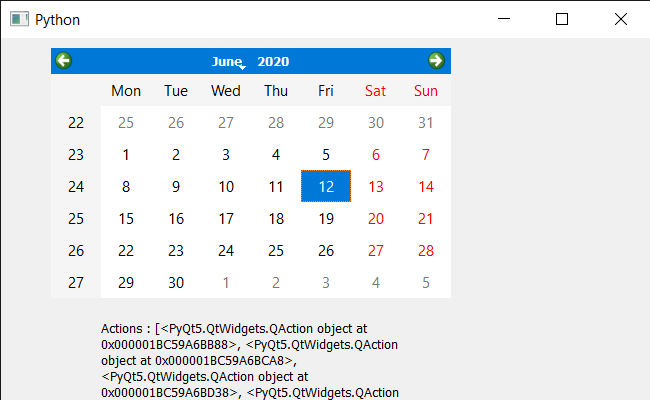Related Articles

# PyQt5 QCalendarWidget – Inserting Multiple QAction

• Last Updated : 15 Jun, 2020

In this article we will see how we can insert multiple QAction to the QCalendarWidget at the same time. In order to do this we use `insertActions` method, this method inserts the action actions to the calendar’s list of actions, before the given action. It appends the actions if before is None or before is not a valid action for this widget.

In order to do this we will use `InsertActions` method with the QCalendarWidget object.

Syntax : calendar.insertActions(before, action_list)

Argument : It takes QAction object and a list as argument

Return : It return None

Below is the implementation

 `# importing libraries``from` `PyQt5.QtWidgets ``import` `*` `from` `PyQt5 ``import` `QtCore, QtGui``from` `PyQt5.QtGui ``import` `*` `from` `PyQt5.QtCore ``import` `*` `import` `sys`` ` `# QCalendarWidget Class``class` `Calendar(QCalendarWidget):`` ` `    ``# constructor``    ``def` `__init__(``self``, parent ``=` `None``):``        ``super``(Calendar, ``self``).__init__(parent)`` ` ` ` `class` `Window(QMainWindow):`` ` `    ``def` `__init__(``self``):``        ``super``().__init__()`` ` `        ``# setting title``        ``self``.setWindowTitle(``"Python "``)`` ` `        ``# setting geometry``        ``self``.setGeometry(``100``, ``100``, ``650``, ``400``)`` ` `        ``# calling method``        ``self``.UiComponents()`` ` `        ``# showing all the widgets``        ``self``.show()`` ` `    ``# method for components``    ``def` `UiComponents(``self``):`` ` `        ``# creating a QCalendarWidget object``        ``# as Calendar class inherits QCalendarWidget``        ``self``.calendar ``=` `Calendar(``self``)`` ` `        ``# setting geometry to the calender``        ``self``.calendar.setGeometry(``50``, ``10``, ``400``, ``250``)`` ` `        ``# setting cursor``        ``self``.calendar.setCursor(Qt.PointingHandCursor)`` ` `        ``# creating a QAction objects``        ``action1 ``=` `QAction(``"Geek"``, ``self``)``        ``action2 ``=` `QAction(``"Python"``, ``self``)`` ` `        ``# creating action list``        ``action_list ``=` `[action1, action2]`` ` `        ``# adding actions to calendar``        ``self``.calendar.addActions(action_list)`` ` `        ``# actions``        ``action_3 ``=` `QAction(``"BTech"``, ``self``)``        ``action_4 ``=` `QAction(``"MTech"``, ``self``)`` ` `        ``# action list``        ``insert_action_list ``=` `[action_3, action_4]`` ` `        ``# inserting action in before second action``        ``self``.calendar.insertActions(action2, insert_action_list)`` ` `        ``# creating label to show the properties``        ``self``.label ``=` `QLabel(``self``)`` ` `        ``# setting geometry to the label``        ``self``.label.setGeometry(``100``, ``280``, ``300``, ``100``)`` ` `        ``# making label multi line``        ``self``.label.setWordWrap(``True``)`` ` `        ``# getting action of the calendar``        ``value ``=` `self``.calendar.actions()`` ` `        ``# setting text to the label``        ``self``.label.setText(``"Actions : "` `+` `str``(value))`` ` ` ` ` ` ` ` `# create pyqt5 app``App ``=` `QApplication(sys.argv)`` ` `# create the instance of our Window``window ``=` `Window()`` ` `# start the app``sys.exit(App.``exec``())`

Output :Attention geek! Strengthen your foundations with the Python Programming Foundation Course and learn the basics.

To begin with, your interview preparations Enhance your Data Structures concepts with the Python DS Course. And to begin with your Machine Learning Journey, join the Machine Learning – Basic Level Course

My Personal Notes arrow_drop_up